Question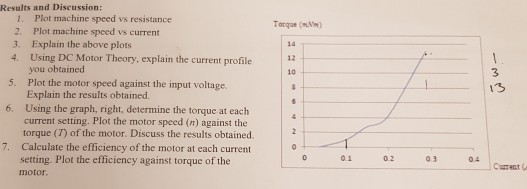DC series MOTOR... LAB

I need help answering question 6 and 7.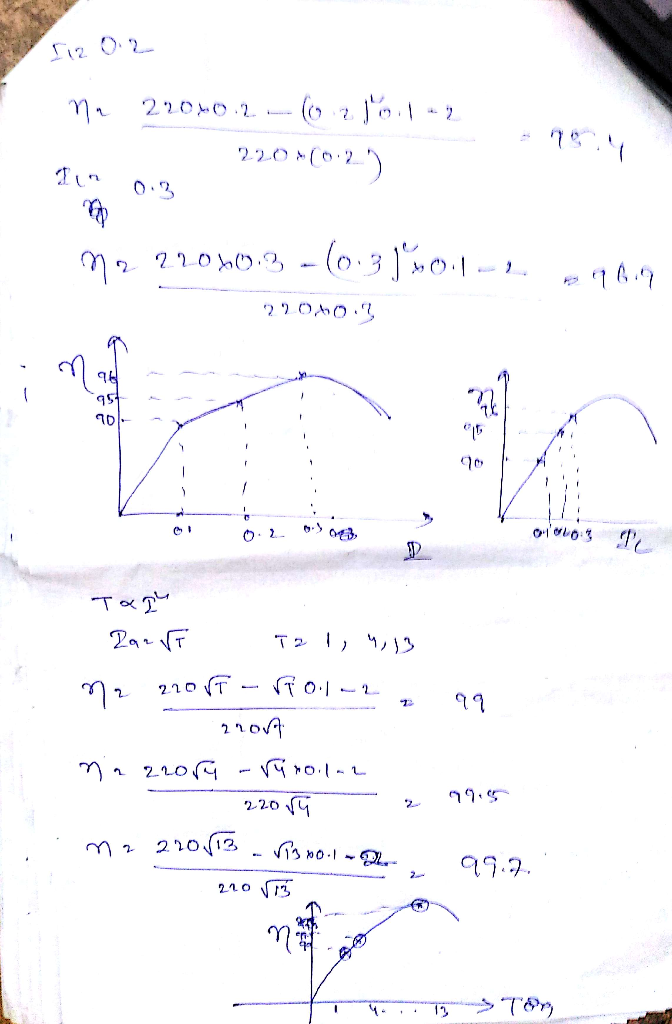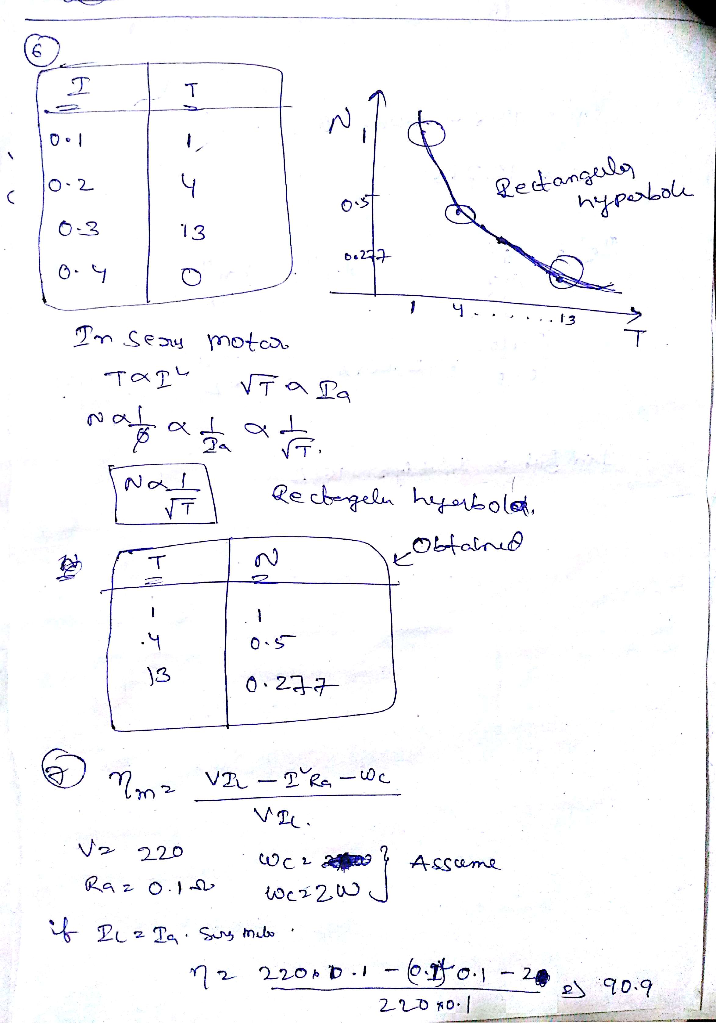#### Earn Coins

Coins can be redeemed for fabulous gifts.

Similar Homework Help Questions
• ### Results and Discussion: 1. Plot machine speed vs resistance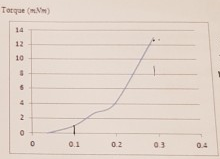DC series MOTOR... LABResults and Discussion: 1. Plot machine speed vs resistance 2. Plot machine speed vs current 3. Explain the above plots 4. Using DC Motor Theory, explain the current profile you obtained 5. Plot the motor speed against the input voltage. Explain the results obtained.  6. Using the graph, right, determine the torque at each current setting. Plot the motor speed (n) against the torque (T) of the motor. Discuss the results obtained.  7. Calculate the efficiency of the motor at each current setting. Plot...

• ### QUESTIONS Question-3: Draw "speed vs. torque" curve using data in Table-1. The completed graph represents the speed vs. torque characteristics of a typical DC shunt-wound motor. Terax Qu...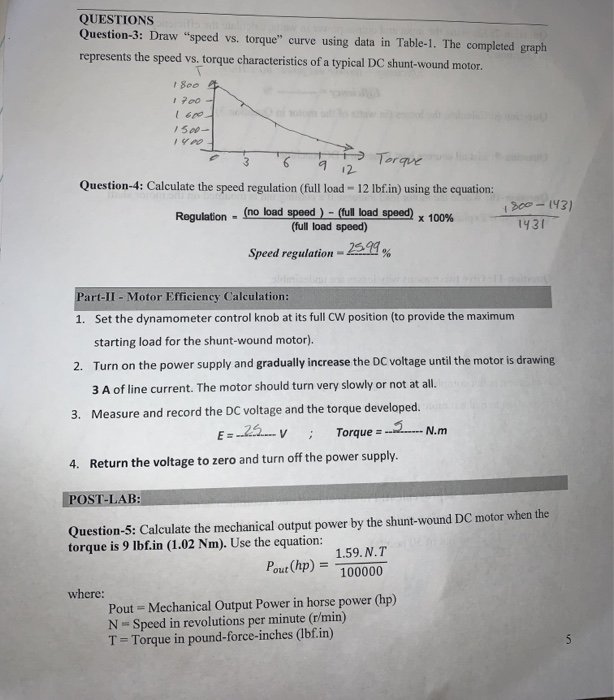QUESTIONS Question-3: Draw "speed vs. torque" curve using data in Table-1. The completed graph represents the speed vs. torque characteristics of a typical DC shunt-wound motor. Terax Question-4: Calculate the speed regulation (full load - 12 lbf.in) using the equation: 2-143) 143 (no load Regulation (full load speed) Speed regulation- % Part-II - Motor Efficiency Calculation: Set the dynamometer control knob at its full CW position (to provide the maximum starting load for the shunt-wound motor). Turn on the power...